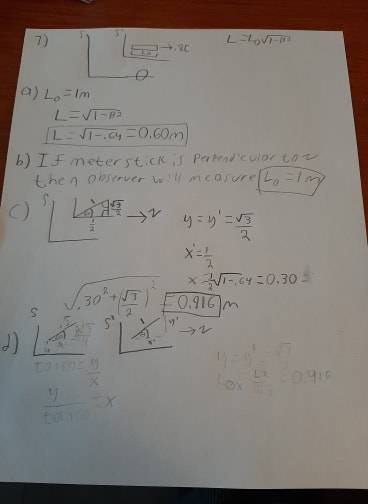# Help with Length contraction of ruler at 60degree angle to observer

dcarmichael
Homework Statement:
7. A meter stick is moving with speed 0.8c relative to a frame S .
(a) What is the stick’s length, as measured by observers in S , if the stick is parallel
to its velocity v ?
(b) What if the stick is perpendicular to v ?
(c) What if the stick is at  60 to v , as seen in the stick’s rest frame? [Hint: You can
imagine that the meter stick is the hypotenuse of a 30-60-90 right triangle]
(d) What if the stick is at  60 to v , as measured in S ?
I believe i have solved a-c , but cant figure out d, looking suggestions?
Relevant Equations:
L=Lo*sqrt (1-β^2)
told to use this equation and not lorentz transformation for this problem